# SBI PO Quantitative Aptitude Questions 2019 (Day-40) High Level New Pattern

Dear Aspirants, the most awaited notification of SBI PO – 2019 has been released. We all know that new pattern questions are introducing every year in the SBI PO exam. Further, the questions are getting tougher and beyond the level of the candidate’s expectations.

Our IBPS Guide is providing High-Level New Pattern Quantitative Aptitude Questions for SBI PO 2019 so the aspirants can practice it on a daily basis. These questions are framed by our skilled experts after understanding your needs thoroughly. Aspirants can practice these high-level questions daily to familiarize with the exact exam pattern. We wish that your rigorous preparation leads you to a successful target of becoming SBI PO.

[WpProQuiz 6227]

### Click Here for SBI PO Pre 2019 High-Quality Mocks Exactly on SBI Standard

Direction (1 – 5): Study the following data carefully and answer the questions:

Pie chart given below shows the percentage distribution of cost price of five items namely P, Q, R, S and T.

Sum of the pie chart is Rs.36000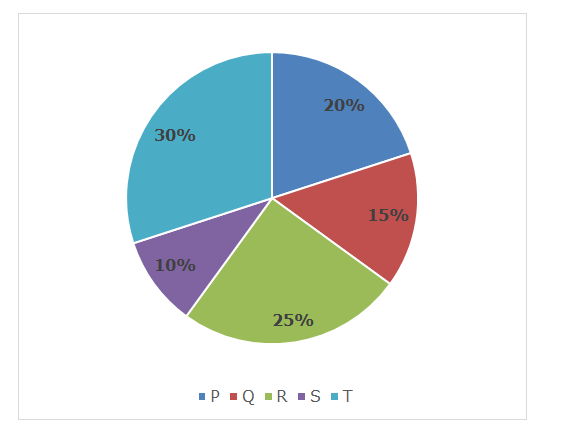Bar graph given below shows the percentage discount offered by shopkeeper A and shopkeeper B on the marked price of those five items.

Note: Marked price of an item is not necessarily same for both the shopkeepers.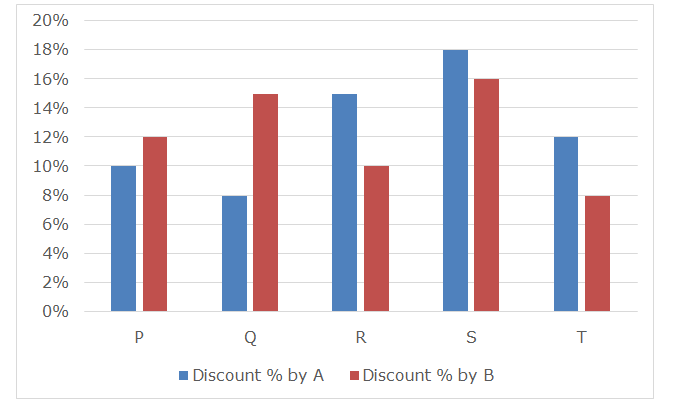1) If marked up price on articles P and Q by shopkeeper A is 35% and 15% respectively and he sold these 2 articles at ‘x’% and ‘y’% profit respectively, then what is the difference between the value of ‘x’ and the value of ‘y’?

a) 14.8

b) 15.7

c) 15.4

d) 13.5

e) None of these

2) Shopkeeper A marked up the price of item P as ‘x’% above its cost price and sold the article at ‘x – 13’% while shopkeeper B marked up the price of item P as ‘y’% above its cost price and sold the article at ‘y – 15’%, then what is the sum of profit amount earned by both the shopkeepers together after selling item P?

a) Rs.1260

b) Rs.1520

c) Rs.1390

d) Rs.1944

e) None of these

3) Marked up amount on item Q by shopkeeper A is half of that by shopkeeper B and sum of profit amount earned by both the shopkeepers together after selling item Q is Rs.330, then what is the ratio amount of discount offered by shopkeeper A to that by shopkeeper B after selling item Q?

a) 7: 13

b) 16: 33

c) 15: 8

d) 26: 63

e) None of these

4) If ratio of marked up amount of item T for shopkeeper A to that for shopkeeper B is 7: 6 and amount of discount offered by shopkeeper A is Rs.648 more than that of  shopkeeper B, then what is the approximate profit per cent earned by both the shopkeepers together after selling item T?

a) 22.44%

b) 66.66%

c) 33.33%

d) 55.55%

e) 28.82%

5) Shopkeeper B sold items R and S to a customer but instead of allowing single cumulative discount he allows two successive discounts on both the items. Discounts offered on item R are 5% and 20% respectively while discounts offered on item S are Rs.220 and 15% respectively and marked up per cent on items R and S are 40% and 45% respectively, then what is the total profit amount earned by the shopkeeper after selling both the items to the customer?

a) Rs.1264

b) Rs.1148

c) Rs.1226

d) 1072

e) None of these

Direction (6 – 10): Study the following data carefully and answer the questions:

There are two cities A and B in a state. A bird flies from city A to city B in the morning for its food and comes back to city A in the evening on five different days of a week starting from Monday to Friday.

Note 1: Flight speed (without wind) of bird from city A to city B in the morning is different on all the five days while its flight speed (without wind) from city B to city A in the evening is also different on all the five days.

2: Direction of wind remains same and is from city B to city A. Speed of wind in the morning is 50 km/h while the speed of wind in the evening is 40 km/h.

First pie chart given below shows the per cent distribution of flight speed (without wind) of bird from city A to city B in the morning on all the five days and sum of the pie chart is 1000 km/h.

Second pie chart given below shows the per cent distribution of flight speed (without wind) of bird from city B to city A in the evening on all the five days and sum of the pie chart is 800 km/h.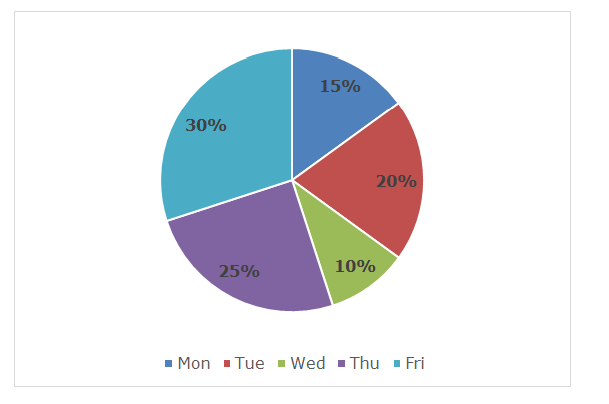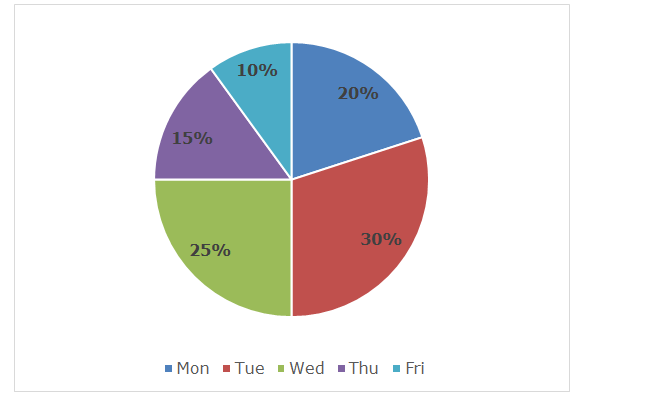6) If the distance between cities A and B is 270 km, then what is the difference between time taken by the bird to reach at city B from city A on Monday in morning and time taken by the bird reach at city A from city B on Friday in evening?

a) 36 minutes

b) 18 minutes

c) 45 minutes

d) 27 minutes

e) None of these

7) On Wednesday morning, the bird starts from city A towards city B and at the same time another bird with its flight speed (without wind) 120 km/h starts from city B towards city A. If difference between total distance travelled by both the bird before meeting is 90 km, then what is the distance between cities A and B?

a) 110 km

b) 220 km

c) 165 km

d) 198 km

e) None of these

8) If the bird starts flying from city A towards city B at 6:00 AM in the morning on Friday and search for food in the city. In the process of searching food it spends 7 hours 9 minutes there and comes back to the city A at 3:00 PM in the evening on the same day, then how far city B is from city A? Assume there is no other loss of time other than flight and searching.

a) 150 km

b) 120 km

c) 180 km

d) 135 km

e) None of these

9) If distance between cities A and B is 98 km and on Tuesday evening the speed of wind is increased by 20 km/h and the bird also increases its flight (without wind) speed while returning from B towards A. Now, time taken by bird to reach at A from B is decreased by 4.2 minutes than the calculated time, then what is the increment in the flight (without wind) speed of bird?

a) 40 km/h

b) 50 km/h

c) 25 km/h

d) 35 km/h

e) None of these

10) If on Thursday, bird flies from city A to city B in the morning but with its flight speed (without wind) 20% more than its original flight (without wind) speed and comes back to the original point in the evening on the same day with speed along the wind as mention in the charts, then how much less time the bird will take then the original time in its flight if cities A and B are 80 km apart?

a) 3.2 minutes

b) 5.4 minutes

c) 4.8 minutes

d) 3.6 minutes

e) None of these

Directions (1 – 5):

Cost price of item P = 20% of 36000 = Rs.7200

Cost price of item Q = 15% of 36000 = Rs.5400

Cost price of item R = 25% of 36000 = Rs.9000

Cost price of item S = 10% of 36000 = Rs.3600

Cost price of item T = 30% of 36000 = Rs.10800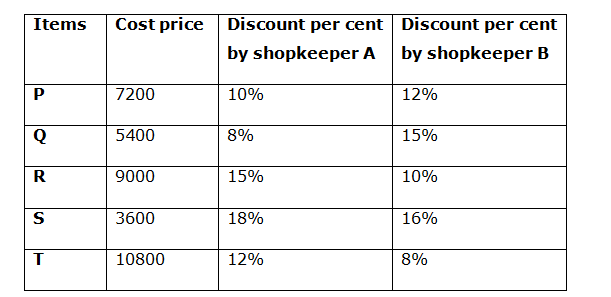Marked price of article P for shopkeeper A = 135% of 7200 = Rs.9720

Selling price of article P for shopkeeper A = 90% of 9720 = Rs.8748

Profit amount earned on article P by shopkeeper A = 8748 – 7200 = Rs.1548

Marked price of article Q for shopkeeper A = 115% of 5400 = Rs.6210

Selling price of article P for shopkeeper A = 92% of 6210 = Rs.5713.2

Profit amount earned on article Q by shopkeeper A = 5713.2 – 5400 = Rs.313.2

According to the question:

x% = (1548/7200) * 100

x% = 21.5%

x = 21.5

y% = (313.2/5400) * 100

y% = 5.8%

y = 5.8

Required difference = x ~ y = 21.5 – 5.8 = 15.7

Marked price of item P for shopkeeper A = (100 + x)% of 7200 = 72(100 + x)

Selling prove of item P for shopkeeper A = 90% of 72(100 + x)

Given that, 90% of 72(100 + x) = (100 + x – 13)% of 7200

64.8(100 + x) = 72(87 + x)

0.9(100 + x) = (87 + x)

90 + 0.9x = 87 + x

3 = 0.1x

x = 30

Profit amount earned by shopkeeper A after selling item P = (x – 13)% of 7200 = 17% of 7200 = Rs.1224

Marked price of item P for shopkeeper B = (100 + y)% of 7200 = 72(100 + y)

Selling prove of item P for shopkeeper B = 88% of 72(100 + y)

Given that, 88% of 72(100 + y) = (100 + y – 15)% of 7200

63.36(100 + y) = 72(85 + y)

0.88(100 + y) = 85 + y

88 + 0.88y = 85 + y

3 = 0.12y

y = 25

Profit amount earned by shopkeeper b after selling item P = (y – 15)% of 7200 = 10% of 7200 = Rs.720

Sum of profit amount earned by both the shopkeepers together after selling item P = 1224 + 720 = Rs.1944

Let marked up amount on item Q by shopkeepers A and B is ‘x’ and ’2x’ respectively.

Marked price of item Q for shopkeeper A = (5400 + x)

Selling price of item Q for shopkeeper A = 92% of (5400 + x) = (4968 + 0.92x)

Profit amount = (4968 + 0.92x) – 5400 = (0.92x – 432)

Marked price of item Q for shopkeeper B = (5400 + 2x)

Selling price of item Q for shopkeeper B = 85% of (5400 + 2x) = (4590 + 1.7x)

Profit amount = (4590 + 1.7x) – 5400 = (1.7x – 810)

According to the question:

(0.92x – 432) + (1.7x – 810) = 330

2.62x – 1242 = 330

2.62x = 1572

x = 600

Amount of discount offered by shopkeeper A = 8% of (5400 + x) = Rs.480

Amount of discount offered by shopkeeper B = 15% of (5400 + 2x) = Rs.990

Required ratio = 480: 990 = 16: 33

Let marked up amount of item T for shopkeeper A and B is 7x and 6x respectively.

Marked price of item T for shopkeeper A = (10800 + 7x)

Amount of discount offered by shopkeeper A = 12% of (10800 + 7x) = (1296 + 0.84x)

Marked price of item T for shopkeeper B = (10800 + 6x)

Amount of discount offered by shopkeeper B = 8% of (10800 + 6x) = (864 + 0.48x)

According to the question:

(1296 + 0.84x) – (864 + 0.48x) = 648

432 + 0.36x = 648

0.36x = 216

x = 600

Profit amount earned by shopkeeper A = [88% of (10800 + 7x)] – 10800

= Rs.2400

Profit amount earned by shopkeeper B = [92% of (10800 + 6x)] – 10800

= Rs.2448

Required profit per cent = [(2400 + 2448)/21600] * 100 = 22.44%

Marked price of item R = 140% of 9000 = Rs.12600

Selling price of item R after two successive discounts = 95% of 80% of 12600

= Rs.9576

Marked price of item S = 145% of 3600 = Rs.5220

Selling price of item R after two successive discounts = 85% of (5220 – 220)

= Rs.4250

Total profit amount earned by the shopkeeper

= (9576 + 4250) – (9000 + 3600) = Rs.1226

Directions (6 – 10):

Flight speed of bird (without wind) on Monday from A to B = 15% of 1000

= 150 km/h

Flight speed of bird (without wind) on Tuesday from A to B = 20% of 1000

= 200 km/h

Flight speed of bird (without wind) on Wednesday from A to B = 10% of 1000

= 100 km/h

Flight speed of bird (without wind) on Thursday from A to B = 25% of 1000

= 250 km/h

Flight speed of bird (without wind) on Friday from A to B = 30% of 1000

= 300 km/h

Flight speed of bird (without wind) on Monday from B to A = 20% of 800

= 160 km/h

Flight speed of bird (without wind) on Tuesday from B to A = 30% of 800

= 240 km/h

Flight speed of bird (without wind) on Wednesday from B to A = 25% of 800

= 200 km/h

Flight speed of bird (without wind) on Thursday from B to A = 15% of 800

= 120 km/h

Flight speed of bird (without wind) on Friday from B to A = 10% of 800

= 80 km/h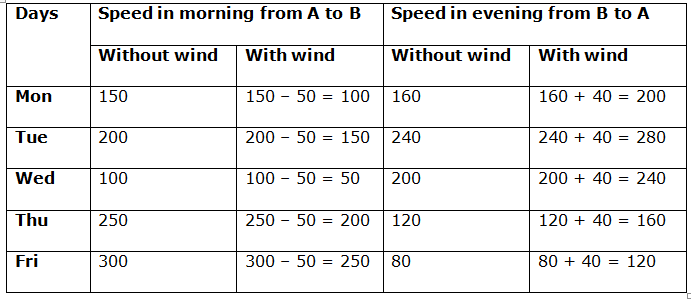Distance between cities A and B = 270 km

Time taken by the bird to reach at city B from city A on Monday in morning

= 270/100 = 2.7 hours

Time taken by the bird reach at city A from city B on Friday in evening

= 270/120 = 2.25 hours

Required difference = 2.7 – 2.25 = 0.45 hours = 27 minutes

Flight speed (without wind) of another bird on Wednesday morning = 120 km/h

Flight speed (with wind) of another bird on Wednesday morning = 120 + 50

= 170 km/h

Effective speed of both the birds = 50 + 170 = 220 km/h

Let both the birds meet after travelling for ‘t’ hours.

Required difference = 170t – 50t = 90

120t = 90

t = 0.75 hours

Distance between both the cities = 220 * 0.75 = 165 km

Time between 6:00 AM in the morning to 3:00 PM in the evening on the same day

= 9 hours = 540 minutes

Time taken in search of food = 7 hours 9 minutes = (7 * 60) + 9 = 429 minutes

Time taken in flight = 540 – 429 = 111 minutes

Let the distance between cities A and B is ‘D’ km.

According to the question:

(D/250) + (D/120) = (111/60)

(12D + 25D)/3000 = 111/60

37D = (111/60) * 3000

37D = 5550

D = 150

Hence, distance between cities A and B is 150 km.

Let increment in the flight speed of bird = ‘x’ km/h

Effective speed of bird on Tuesday evening while returning from B to A

= (280 + 20 + x) = (300 + x) km/h

Calculated time taken by bird = (98/280) * 60 = 21 minutes

Actual time taken by bird = (21 – 4.2) = (98 * 60)/(300 + x)

300 + x = 350

x = 50

Increment in the flight (without wind) speed of bird = x = 50 km/h

Changed flight speed (without wind) of the bird = 120% of 250 = 300 km/h

Changed flight speed (with wind) of the bird = 300 – 50 = 250 km/h

Original flight time of the bird = (80/200) + (80/160) = 0.4 + 0.5 = 0.9 hours

= 54 minutes

Original flight time of the bird = (80/250) + (80/160) = 0.32 + 0.5 = 0.82 hours

= 49.2 minutes

Required less time = 54 – 49.2 = 4.8 minutes